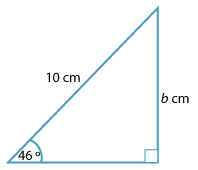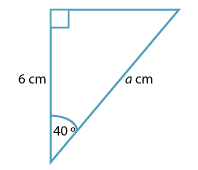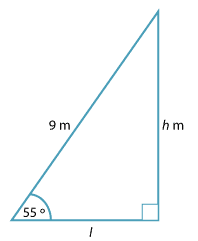#### Question 1Detailed description

 a b The ratio $$\dfrac{b}{10}$$ = sin 46° cos 46° tan 46° The value of b correct to 4 decimal places is? 7.0711 10.3553 7.1934

#### Question 2Detailed description

The value of a can be found by solving the equation.

 a $$a = 6 \ \text{cos}\ 40°$$ $$a = \dfrac{\text{cos}\ 40°}{6}$$ $$a = \dfrac{6}{\text{cos}\ 40°}$$

#### Question 3

The diagram is of a ladder 9 m long resting against a wall, making an angle of 55° with the ground.Detailed description

a b The height h that the ladder reaches up the wall, correct to the nearest centimetre, is: 5.16 m 7.37 m 6.30 m The distance l from the foot of the ladder to the wall, correct to the nearest centimeter, is: 5.16 m 7.37 m 6.30 m